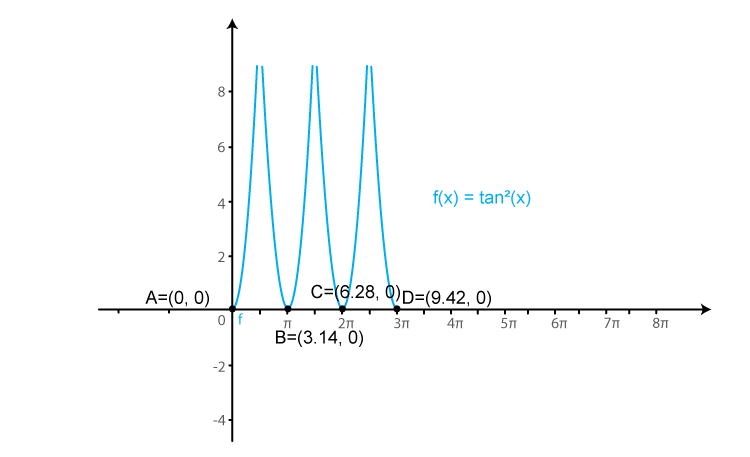# RD Sharma Solutions for Class 11 Chapter 6 - Graphs of Trigonometric Functions Exercise 6.3

In Exercise 6.3, we shall study problems based on the graph of tangent, cosecant, cotangent and secant functions. Students who are finding difficulty in solving problems of this chapter can download the RD Sharma Class 11 Solutions pdf which is prepared by subject experts at BYJU’S who have vast academic experience. Here, the students can access the RD Sharma Solutions for Class 11 Maths Chapter 6 free pdf to improve their speed in solving problems as well as boost their confidence level before taking the final board exam.

## Download the Pdf of RD Sharma Solutions for Class 11 Maths Exercise 6.3 Chapter 6 – Graphs of Trigonometric Functions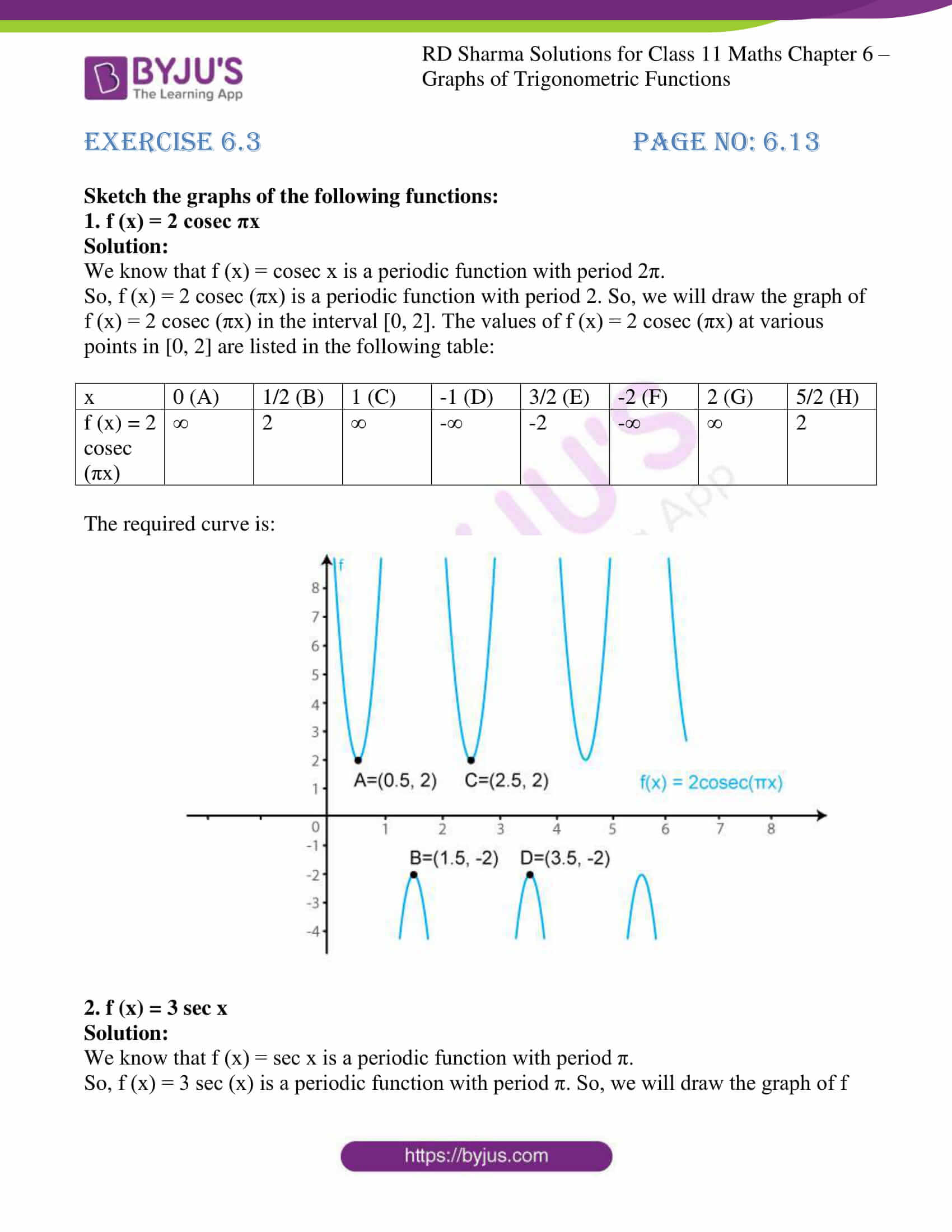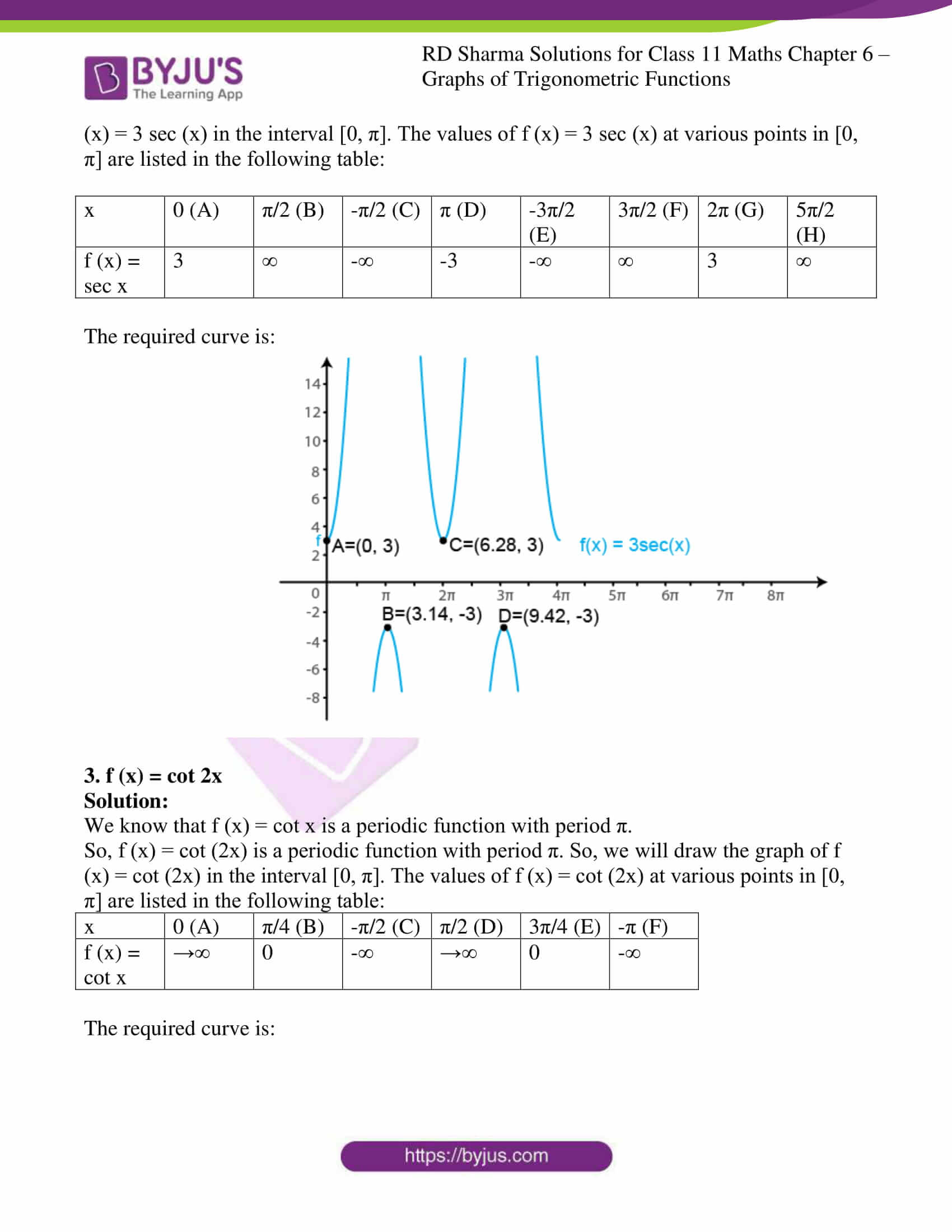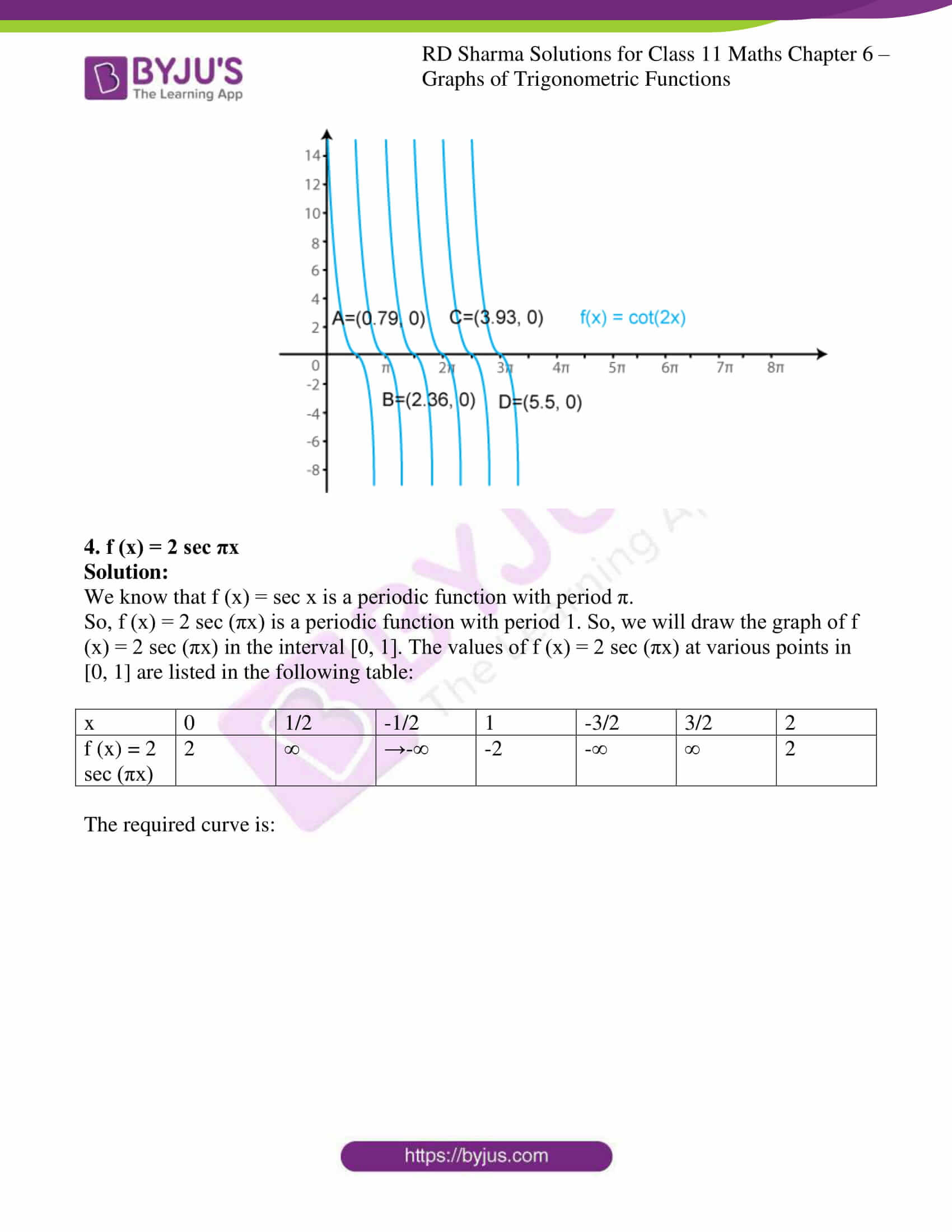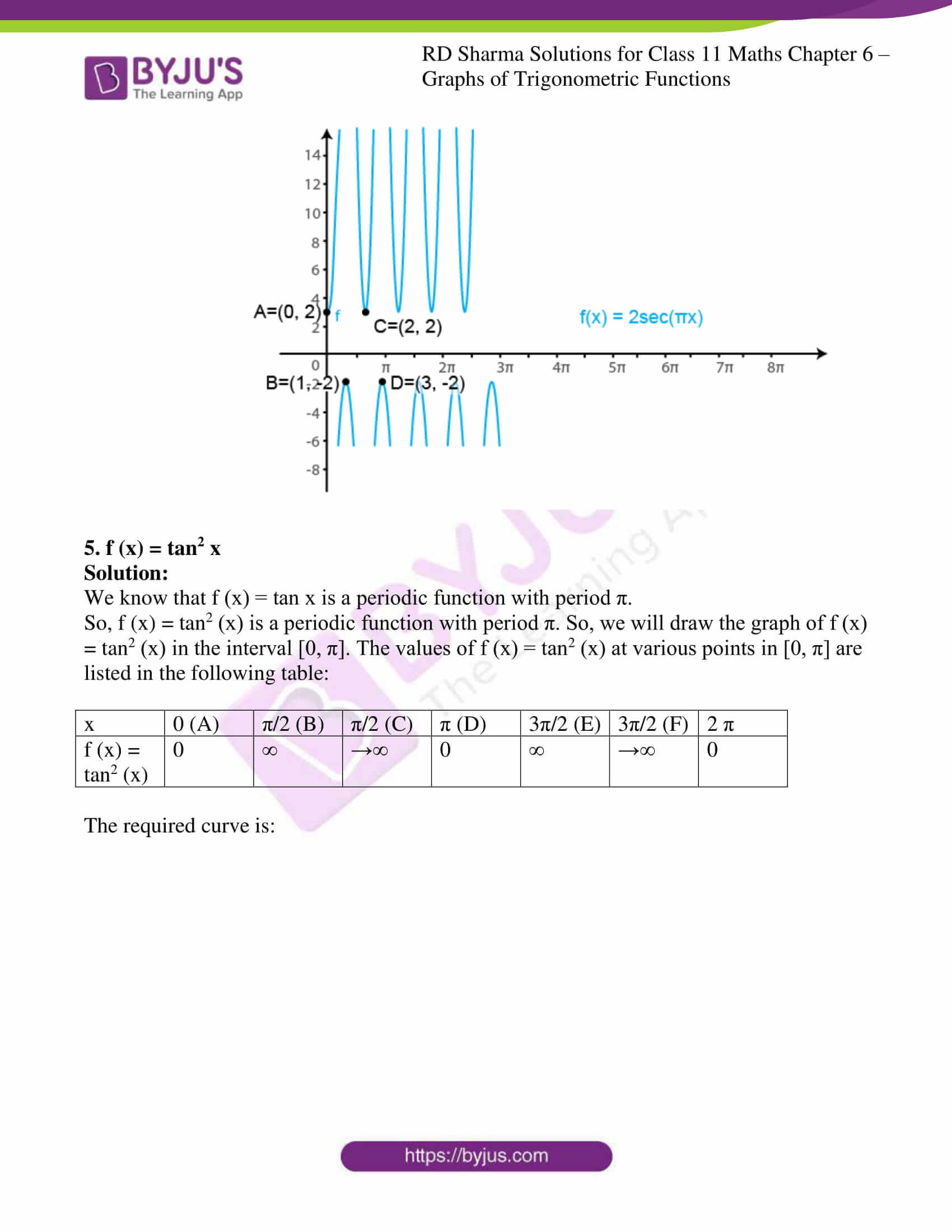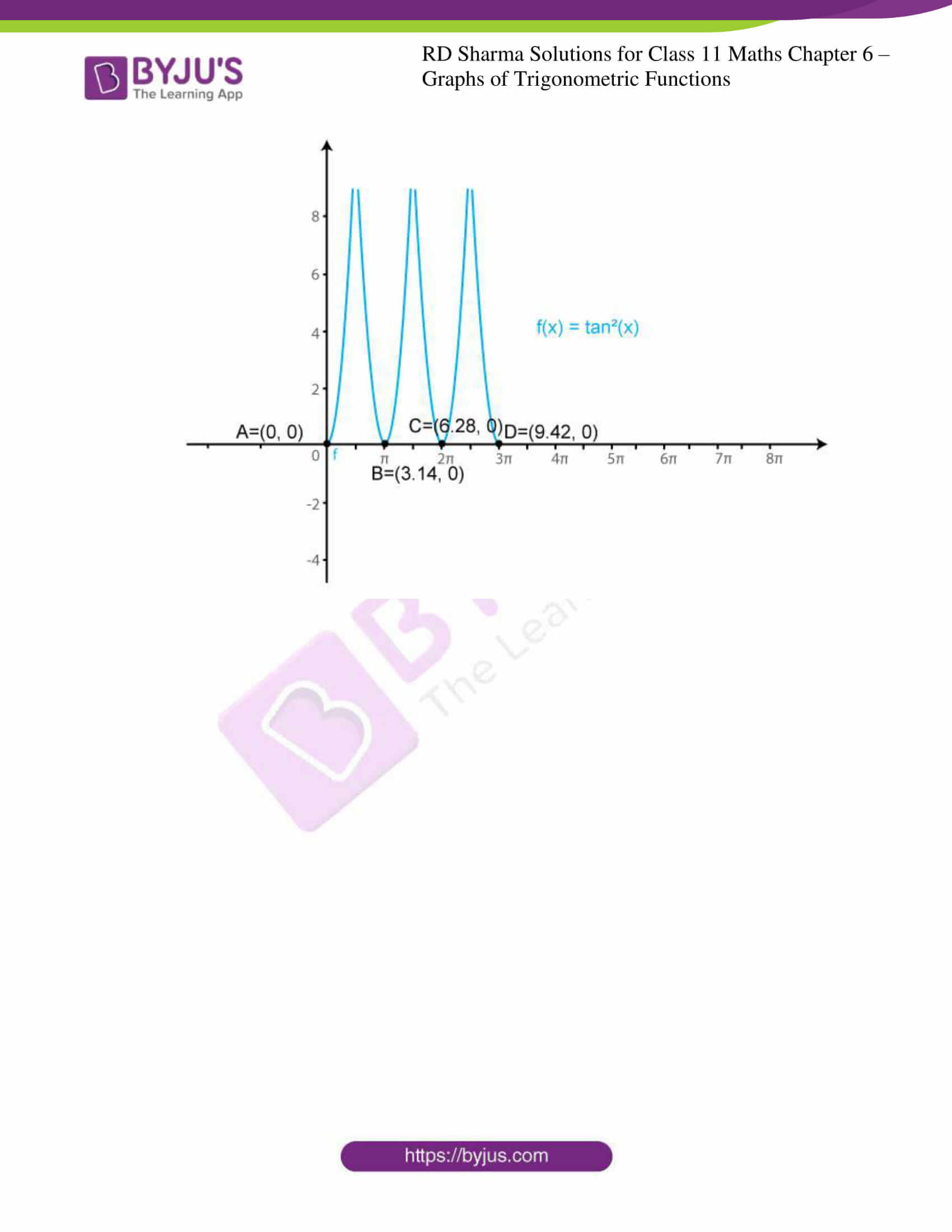### Access answers to RD Sharma Solutions for Class 11 Maths Exercise 6.3 Chapter 6 – Graphs of Trigonometric Functions

Sketch the graphs of the following functions:

1. f (x) = 2 cosec πx

Solution:

We know that f (x) = cosec x is a periodic function with period 2π.

So, f (x) = 2 cosec (πx) is a periodic function with period 2. So, we will draw the graph of f (x) = 2 cosec (πx) in the interval [0, 2]. The values of f (x) = 2 cosec (πx) at various points in [0, 2] are listed in the following table:

 x 0 (A) 1/2 (B) 1 (C) -1 (D) 3/2 (E) -2 (F) 2 (G) 5/2 (H) f (x) = 2 cosec (πx) ∞ 2 ∞ -∞ -2 -∞ ∞ 2

The required curve is: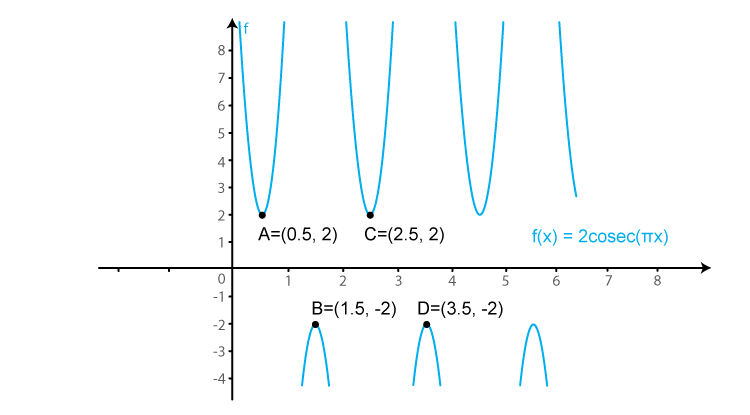2. f (x) = 3 sec x

Solution:

We know that f (x) = sec x is a periodic function with period π.

So, f (x) = 3 sec (x) is a periodic function with period π. So, we will draw the graph of f (x) = 3 sec (x) in the interval [0, π]. The values of f (x) = 3 sec (x) at various points in [0, π] are listed in the following table:

 x 0 (A) π/2 (B) -π/2 (C) π (D) -3π/2 (E) 3π/2 (F) 2π (G) 5π/2 (H) f (x) = sec x 3 ∞ -∞ -3 -∞ ∞ 3 ∞

The required curve is: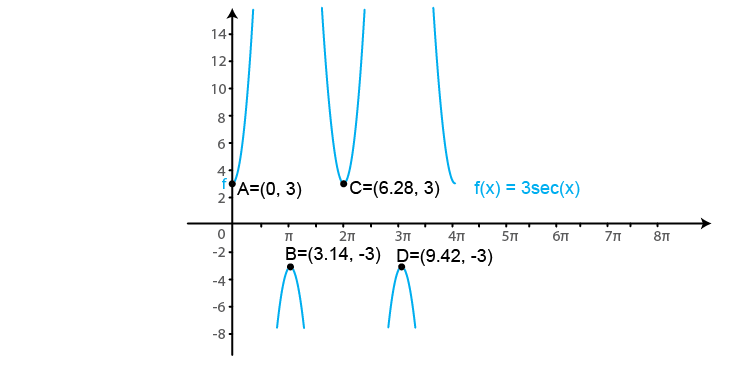3. f (x) = cot 2x

Solution:

We know that f (x) = cot x is a periodic function with period π.

So, f (x) = cot (2x) is a periodic function with period π. So, we will draw the graph of f (x) = cot (2x) in the interval [0, π]. The values of f (x) = cot (2x) at various points in [0, π] are listed in the following table:

 x 0 (A) π/4 (B) -π/2 (C) π/2 (D) 3π/4 (E) -π (F) f (x) = cot x →∞ 0 -∞ →∞ 0 -∞

The required curve is: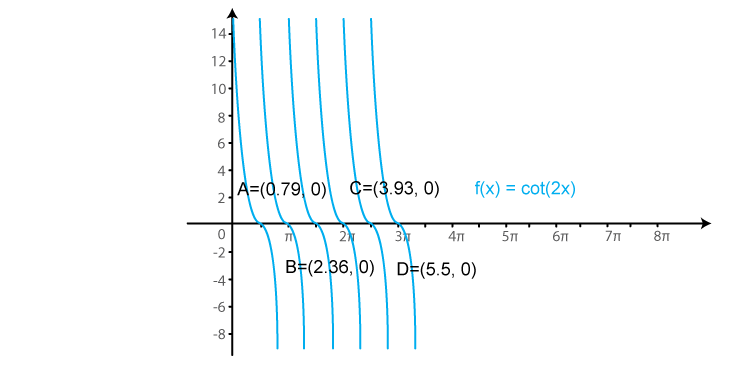4. f (x) = 2 sec πx

Solution:

We know that f (x) = sec x is a periodic function with period π.

So, f (x) = 2 sec (πx) is a periodic function with period 1. So, we will draw the graph of f (x) = 2 sec (πx) in the interval [0, 1]. The values of f (x) = 2 sec (πx) at various points in [0, 1] are listed in the following table:

 x 0 1/2 -1/2 1 -3/2 3/2 2 f (x) = 2 sec (πx) 2 ∞ →-∞ -2 -∞ ∞ 2

The required curve is: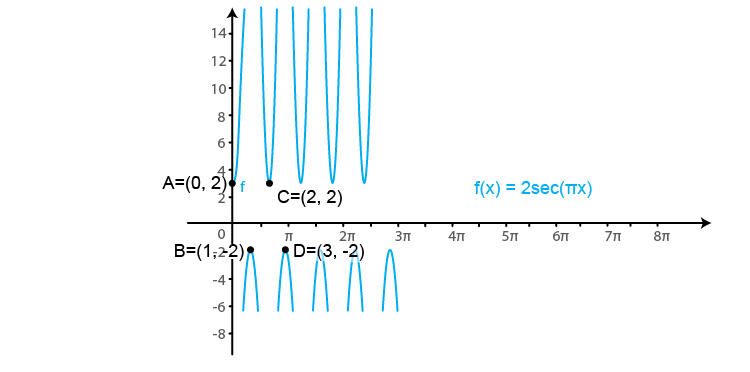5. f (x) = tan2 x

Solution:

We know that f (x) = tan x is a periodic function with period π.

So, f (x) = tan2 (x) is a periodic function with period π. So, we will draw the graph of f (x) = tan2 (x) in the interval [0, π]. The values of f (x) = tan2 (x) at various points in [0, π] are listed in the following table:

 x 0 (A) π/2 (B) π/2 (C) π (D) 3π/2 (E) 3π/2 (F) 2 π f (x) = tan2 (x) 0 ∞ →∞ 0 ∞ →∞ 0

The required curve is: